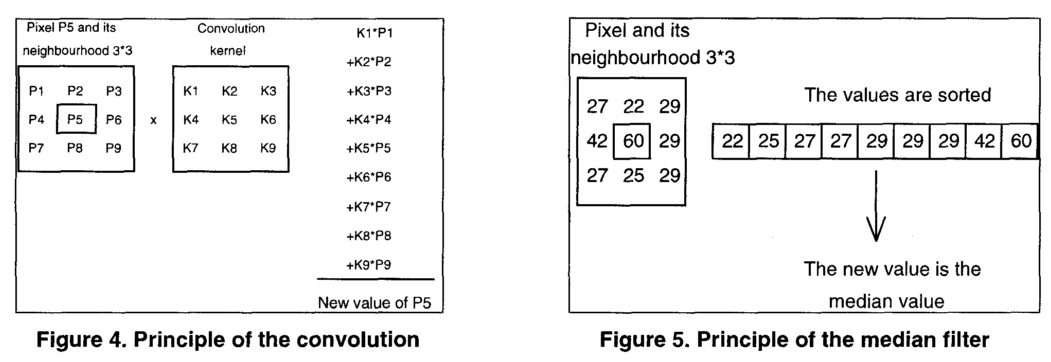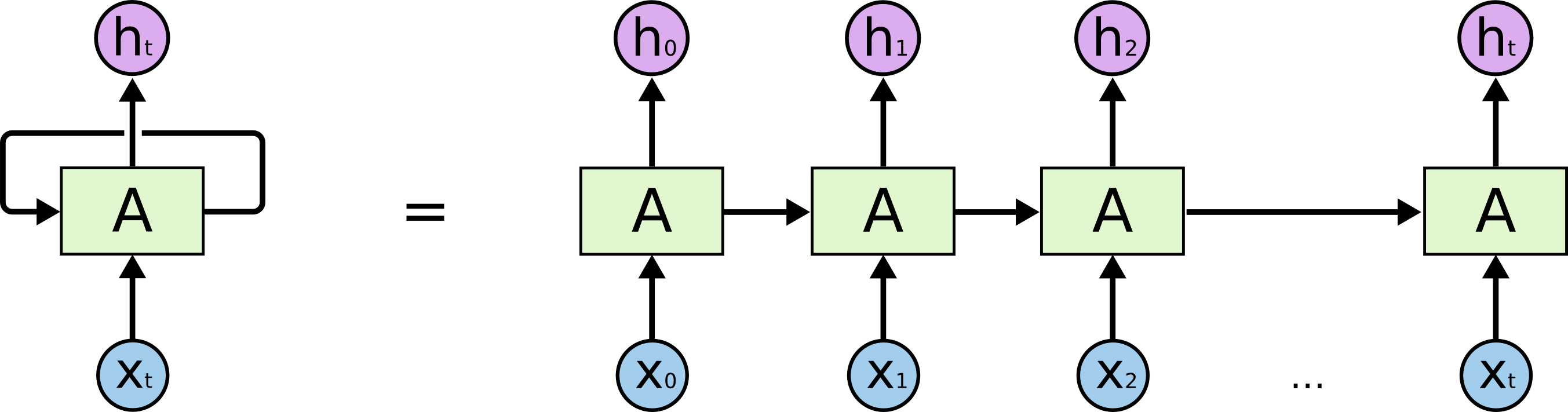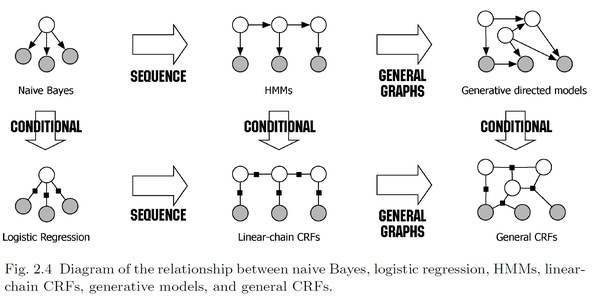# 裂缝识别

## Introduction

• 会出现孤立“噪点”————与裂缝特征相近的单个小块被错误识别为裂缝。由于工程应用中对裂缝的定义是连续线条，这些噪点会对最后的结果带来误差。
• 此外，有些裂纹会在中间一段不明显，CNN方法不会将这部分识别为裂缝，而工程应用恰恰需要把它们连成一体。
• 通过单一的卷积神经网络的方法对病害中的裂缝和条形修补进行识别，在路况良好（无光照阴影，路面清洁，老化不严重）的路上识别的准确率可以达到90%以上，但是对路况普通（存在光照等问题，也存在较好路况）的路面，效果一般，准确率只能达到70%左右。

Conditional Random Fields(CRF)用于pixel-wise的图像标记（其实就是图像分割）。把像素的label作为形成马尔科夫场随机变量且能够获得全局观测时，CRF便可以对这些label进行建模。这种全局观测通常就是输入图像。即在判断某一个方格时需要考虑整张图的情况，从而解决“噪点”和连接的问题。• Contribution：这篇论文最重要的工作就是end-to-end训练。CNN负责产生unary potential，intuitively上更合理，实验效果也证明有提高。具体通过两方面来实现：
• mean-field CRF 的RNN实现：将mean-field算法的步骤分解为CNN，这样通过迭代就成了RNN。
• error differential 的反向传递，CRF的error derivative直接传到了CNN。
• 关键问题：将 label assignment problem 转化为 probability inference problem ，融入对相似像素的标签一致性的推断。
• 目标：从 coarse 到 fine-grained segmentation ，弥补 CNN 在 pixel-level labeling task 上的不足。

## 1. 理论部分

### Feature Extraction : CNN##

• 卷积：Conv2D 维数不变
• 池化：Maxpooling2D 缩小维数
• pool_size
• stride

• $B$个 annotation vector，对应$B$个方格
• 每个vector都是$D$维(dimension)的

without considering the smoothness and consistency of the label assignment. 因此需要考虑pairwise data-dependant smoothing term. 作为CRF的一元势能量。

• receptive field
• subsampling rate

### 关于RNN### 关于CRF(概率图模型)• $X$：由$X_1,X_2,...,X_N$组成的随机向量；
• $N$就是图像的像素个数。

 $Z(I)$是所有可能labeling的集合，保证$$P(X=x I)$$在0~1。

#### Recall the HMM :

(transition probability x emission probability)。## 2. 网络构建

• 结构：
• CNN :unary potential： $\varphi_u(x_i)$
• CRF : pairwise potential as weighted Gaussians.

$\mathbf f_i$是像素$i$的feature vector，这里是从原图片中提取的。 Potts Model$\mu(x_i,x_j)=[x_i \neq x_j]$ 是对位置接近的相似像素标记不同的惩罚项。

• inference method
• back propagation : 论文中使用的是SGD来进行反向传播；
• minibatch ：训练时可以用一整张图，也可以用多张图。

### Mean-field = CNN + softmax

#### 2. Message passing:

$Q$进行$M$个高斯滤波：

• Gaussian kernel
• edge-preserving Gaussian filters
• coefficient直接从像素位置、RGB信息中来，反映和其他像素的关联程度
• 这里的CRF是potentially fully connected ，每个filter的receptive field都是整张图，强制实现不可行。
• 为了提高高位高斯铝箔的速度，使用的是Andrew Adams et al所提出的Pemutohedral Lattice，时间复杂度$O(n)$• 分为三个步骤：
• splat
• blur
• slice
• 误差反向通过filter，逆转blur阶段filter的顺序，时间复杂度$O(n)$

• 2 Guassian Kernel：Bilateral filter 和Spatial filter两种滤波器

#### 4. Compatibility Transform

• $\mu (l,l')$：两个标签之间的compatibility
• 如果相似像素标签不同就有 penalty。Intuitively,不同的 label pair 有不同的penalty会合理一些。
• 同样可看作是卷积操作，且输入输出都有$L$个channel，学习filter的参数就是在学习函数$\mu(.,.)$

#### 5. unary-compatibility transform

• 未引入其他参数，误差直接传递给两个输入，注意符号

#### 6. Normalization：softmax

 计算$$P(\mathbf X=\mathbf x \mathbf I)$$：
• softmax的反向传播

### Iterable Mean-field = RNN

• $Q_{in}$：an estimation marginal probability from the previous iteration:

• t=0,使用softmax（U）来初始化
• t>1,每轮$f_{\theta}$中的$Q_{in}$为上一轮输出
• 输出$Y$
• 学习算法：BP through time
• 5次迭代即可收敛，再增加可能导致vanishing和exploding gradient problems。
实现Bilateral filter 的bilateral_lattices_和实现Spatial filter的spatial_lattice

## 3. Caffe上的实现

Caffe 是由伯克利大学视觉和学习中心开发的一种深度学习框架。在视觉任务和卷积神经网络模型中，Caffe 格外受欢迎且性能优异。

$x=\{x_1,\dots,x_P\}$ $P$为像素数。

### 自己写Python层作为输入层

road_layer.py

self.x = np.load(os.path.join(CACHE_PATH, 'x_{}.npy'.format(self.split)))
self.y = np.load(os.path.join(CACHE_PATH, 'y_{}.npy'.format(self.split)))

• setup()是类启动时该做的事情，比如层所需数据的初始化。
• reshape()就是取数据然后把它规范化为四维的矩阵。每次取数据都会调用此函数。
• forward()就是网络的前向运行，这里就是把取到的数据往前传递，因为没有其他运算。
• assign output
• pick next input
• backward()就是网络的反馈，data层是没有反馈的，所以这里就直接pass。

### 生成训练网络

net.py生成网络结构文件

    n.data, n.label = L.Python(module='road_layer', layer='ROADSegDataLayer',
ntop=2, param_str=str(pydata_params))

# the base net
n.conv1_1, n.relu1_1 = conv_relu(n.data, 32, ks=5)
n.conv1_2, n.relu1_2 = conv_relu(n.relu1_1, 32, ks=5)
n.pool1 = max_pool(n.relu1_2)

n.conv2_1, n.relu2_1 = conv_relu(n.pool1, 64)
n.conv2_2, n.relu2_2 = conv_relu(n.relu2_1, 64)
n.pool2 = max_pool(n.relu2_2)

n.conv3_1, n.relu3_1 = conv_relu(n.pool2, 128)
n.conv3_2, n.relu3_2 = conv_relu(n.relu3_1, 128)
n.pool3 = max_pool(n.relu3_2)

n.conv4_1, n.relu4_1 = conv_relu(n.pool3, 256)
n.conv4_2, n.relu4_2 = conv_relu(n.relu4_1, 256)
# n.conv4_3, n.relu4_3 = conv_relu(n.relu4_2, 512)
n.pool4 = max_pool(n.relu4_2)

n.conv5_1, n.relu5_1 = conv_relu(n.pool4, 256)
n.conv5_2, n.relu5_2 = conv_relu(n.relu5_1, 256)
# n.conv5_3, n.relu5_3 = conv_relu(n.relu5_2, 512)
n.pool5 = max_pool(n.relu5_2)

n.score = conv(n.pool5, 1)
n.loss = L.SigmoidCrossEntropyLoss(n.score, n.label)
# n.loss = L.MultiStageMeanfield()


### Solver 优化

• 选择合适的learning rate比较困难
• 对所有的参数更新使用同样的learningrate。对于稀疏数据或者特征，有时我们可能想更新快一些对于不经常出现的特征，对于常出现的特征更新慢一些，这时候SGD就不太能满足要求了
• SGD容易收敛到局部最优，并且容易被困在鞍点train_net: "train.prototxt"
# train_net: "TVG_CRFRNN_new_deploy.prototxt"
test_net: "val.prototxt"
test_iter: 16
test_interval: 500
base_lr: 5e-4
lr_policy: "fixed"
momentum: 0.99
type: "Adam"
weight_decay: 0.0005
display: 100
max_iter: 1000
snapshot: 1000
snapshot_prefix: "snapshot/train"
solver_mode: GPU


This parameter indicates how the learning rate should change over time. This value is a quoted string.

1. Options include:
• “step” - drop the learning rate in step sizes indicated by the gamma parameter.
• “multistep” - drop the learning rate in step size indicated by the gamma at each specified stepvalue.
• “fixed” - the learning rate does not change.
• “exp” - gamma^iteration
• “poly” -
• “sigmoid” -

### 训练caffemodel

../CRFasRNN-merge/caffe-master/build/tools/caffe train -solver solver.prototxt


solver = caffe.SGDSolver('models/bvlc_reference_caffenet/solver.prototxt')


### 整合crfasrnn

net.params : a vector of blobs for weight and bias parameters

net.params['conv1_1'] contains the weight parameters, an array of shape (32, 1, 5, 5) net.params‘conv’ contains the bias parameters, an array of shape (3,)

solver = caffe.SGDSolver('solver.prototxt')
solver.net.copy_from('pretrained_param_file')
[...]

## control layer's initialization
halt_training = False
for layer in solver.net.params.keys():
for index in range(0, 2):
if len(solver.net.params[layer]) < index+1:
continue

if np.sum(solver.net.params[layer][index].data) == 0:
print layer + ' is composed of zeros!'
halt_training = True

if halt_training:
print 'Exiting.'
exit()


To launch one step of the gradient descent, that is a forward propagation, a backward propagation and the update of the net params given the gradients (update of the net.params[k][j].data) :

solver.step(1)


## 4. 细节

### Loss Function

• 训练时迭代5，测试时迭代10
• Loss function：
• 训练时：log likelihood
• 测试时：average IU

average intersection over union(IU)

### Normalization techniques

rectified linear unit (ReLU)

## 5. 环境搭建

    sudo pip install -r requirements.txt


### 测试

    #!/bin/bash

TOOLS=../../caffe/build/tools
WEIGHTS=TVG_CRFRNN_COCO_VOC.caffemodel
SOLVER=TVG_CRFRNN_new_solver.prototxt

$TOOLS/caffe train -solver$SOLVER -weights \$WEIGHTS -gpu 0


### Debug

1. cuda
    libcudart.so.7.0: cannot open shared object file: No such file or directory

    sudo ldconfig /usr/local/cuda-7.5/lib64

1. Python
    Unknown layer type: Python (known types: AbsVal, Accuracy, ArgMax, BNLL, Concat, ContrastiveLoss, Convolution, Crop, Data, Deconvolution, Dropout, DummyData, Eltwise, Embed, EuclideanLoss, Exp, Filter, Flatten, HDF5Data, HDF5Output, HingeLoss, Im2col, ImageData, InfogainLoss, InnerProduct, LRN, Log, MVN, MemoryData, MultiStageMeanfield, MultinomialLogisticLoss, PReLU, Pooling, Power, ReLU, Reduction, Reshape, SPP, Sigmoid, SigmoidCrossEntropyLoss, Silence, Slice, Softmax, SoftmaxWithLoss, Split, TanH, Threshold, Tile, WindowData)


WITH_PYTHON_LAYER := 1


rm -rf ./build/*
make all -j8


8 is the number of parallel threads for compilation (a good choice for the number of threads is the number of cores in your machine).

2.1 build errors:undefined cv::imread() cv::imencode() …

cmake

• Efficient Inference in Fully Connected CRFs with Gaussian Edge Potentials